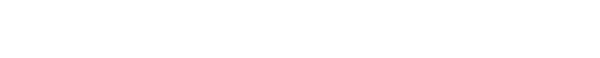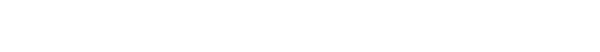●  Jupyter Notebook ：一个对于数据分析师来说特别合适的Python编辑器，强烈推荐大家去使用。
●  Python：在机器学习时代，Python是最受欢迎的机器学习语言。有很多机器学习的库，可以方便高效的去实现机器学习。

●  pandas：是基于 Numpy 构建的含有更高级数据结构和工具的数据分析包。能很方便的进行各种数据清洗。是每个数据分析师必学的Python包之一。
●  sklearn：是机器学习中一个常用的第三方包，里面对一些常用那个的机器学习方法进行了封装，使得大家能够更加简单的使用机器学习的方法。本文主要用这个包进行训练数据集和测试数据集的拆分以及数据尺度的标准化。
●  Keras：是一个高层神经网络API，Keras由纯Python编写而成并基Tensorflow、Theano以及CNTK后端。本文是基于Tensorflow后端构建神经网络模型。Tensorflow是谷歌开发的一个开源的人工智能库。

``````
``````
`#载入pandas包来读取csv格式的数据集`

`import pandas as pd`

`#把 csv格式的数据集导入到DataFrame对象中`

`df = pd.read_csv('C:/Users/36540/Desktop/lossertest.csv', header = 0)`

`df.head()```````
``````
`#把totalPaiedAmount列也就是用户付款金额的缺失值替换为0`

`df['totalPaiedAmount'] = df['totalPaiedAmount'].fillna(0)`

`df['totalBuyCount'] = df['totalBuyCount'].fillna(0)`

``````
``````
`#利用pandas中的to_datetime函数把字符串的日期变为时间序列`

`df['registrationTime'] = pd.to_datetime(df['registrationTime'], format='%Y-%m-%d %H:%M:%S')`

`df['registrationTime']```````
``````
`#同理最近登录时间也转化为实践序列`

`df['lastLoginTime'] = pd.to_datetime(df['lastLoginTime'], format='%Y-%m-%d %H:%M:%S')`

`df['lastLoginTime']```````
``````
`import datetime`

`#获取当前时间`

`now_time = datetime.datetime.now()`

`now_time```````
``````
`#把数据序列转化为距今的时间间隔`

`df['registrationTime'] = now_time-df['registrationTime']`

`df['registrationTime']```````
``````
`df['lastLoginTime'] = now_time-df['lastLoginTime']`

`df['registrationTime']```````
``````
`#把最近登录时间列的空值替换为同索引行注册时间列的值`

`df.loc[df['lastLoginTime'].isnull(),'lastLoginTime']=df[df['lastLoginTime'].isnull()]['registrationTime']`

`df['registrationTime']```````
``````
`#因为数据量有点大，取前1w行数据测试下`

`df = df.iloc[0:1000]`

`#把时间间隔转化为数值型的天数`

`j = 0`

`for i in df['registrationTime']:`

`df = df.replace(df['registrationTime'][j],i.days)`

`j += 1`

``````
``````
`#不知道为什么这样操作就会报错，欢迎大家研究研究`

`for i in range(0,df['registrationTime']):`

`df = df.replace(df['registrationTime'][i],df['registrationTime'][i].days)`

``````
``````
`#对数据集进检查，看看是否还有缺失值`

`df[df.isnull().values==True]```````
``````
`#把第一列无用的用户ID列删除`

`df = df.iloc[:,1:]`

``````
``````
`df.head()`

`df.info()```````
``````
`#把输入输出项确定下`

`y = df.iloc[:,-1]`

`x = df.iloc[:,:-1]`

`x.shape`

`y.shape```````
``````
`#sklearn把数据集拆分成训练集和测试集`

`from sklearn.model_selection import train_test_split`

`x_train, x_test, y_train, y_test = train_test_split(x, y, test_size = 0.33, random_state = 123)`

`x_train.shape`

`y_train.shape`

`x_test.shape`

`y_test.shape```````
``````
`#使用sklearn把数据集进行尺度标准化`

`from sklearn.preprocessing import StandardScaler`

`sc = StandardScaler()`

`x_train = sc.fit_transform(x_train)`

`x_test = sc.fit_transform(x_test)`

`x_test`sklearn包中的StandardScaler函数可以方便对数据进行去均值和方差归一化处理。首先定义一个对象，sc = StandardScaler()，然后把数据集放进去就可以直接输出一个标准化完成的数据集。输出的数据集如上图所示。

``````
``````
`#使用keras包搭建人工神经网络`

`import keras`

`#序贯（Sequential）模型包`

`from keras.models import Sequential`

`#神经网络层`

`from keras.layers import Dense`

`#优化器`

`from keras.optimizers import SGD`

`#创建一个空的神经网络模型`

`classifier = Sequential()`

``````
``````
`#创建输入层`

`classifier.add(Dense(units = 3, kernel_initializer = 'uniform', activation = 'relu', input_dim = 6))`

`#创建输出层`

`classifier.add(Dense(units = 1, kernel_initializer = 'uniform', activation = 'sigmoid'))`

Dense就是常用的全连接层，所实现的运算是`output = activation(dot(input, kernel)+bias)`

●  units：大于0的整数，代表该层的输出维度。一般为输入项的一半，但是真正合适的值还是要经过多次训练才能得出。
●  activation：激活函数，为预定义的激活函数名（参考激活函数），或逐元素（element-wise）的Theano函数。如果不指定该参数，将不会使用任何激活函数（即使用线性激活函数：a(x)=x）。本文用的relu和sigmoid。都是最基础的。
●  bias_initializer：偏置向量初始化方法，为预定义初始化方法名的字符串，或用于初始化偏置向量的初始化器。不同的层可能使用不同的关键字来传递初始化方法，一般来说指定初始化方法的关键字。本文用的Glorot均匀分布初始化方法，又成Xavier均匀初始化，参数从[-limit, limit]的均匀分布产生，其中limit为sqrt(6 / (fan_in + fan_out))。fan_in为权值张量的输入单元数，fan_out是权重张量的输出单元数。

``````
``````
`classifier.compile(loss='binary_crossentropy',`

`optimizer=SGD(),`

`metrics=['accuracy'])`

` `

`history = classifier.fit(x_train, y_train,`

`batch_size=10,`

`epochs=100,`

`validation_data=(x_test, y_test))`Keras以Numpy数组作为输入数据和标签的数据类型。训练模型一般使用fit函数。把训练集输入，然后batch_size选择每次训练数量，epochs是训练的次数。validation_data验证的数据集。

``````
``````
`y_pred = classifier.predict(x_test)`

`y_pred```````
``````
`y_pred = (y_pred > 0.5)`

`y_pred.shape`

`y_pred.flatten().astype(int)```````
``````
`from sklearn.metrics import accuracy_score`

`accuracy_score(y_test, y_pred )```````
``````
`from sklearn.metrics import confusion_matrix`

`cm = confusion_matrix(y_test, y_pred )`

`cm```````
``````
`from sklearn.metrics import classification_report`

`print(classification_report(y_test, y_pred))`